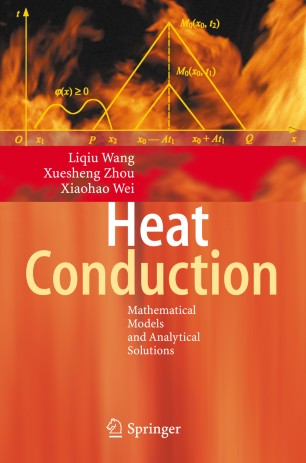# Heat Conduction

## Mathematical Models and Analytical Solutions

• Liqiu Wang
• Xuesheng Zhou
• Xiaohao Wei1. Front Matter
Pages I-XIII
2. Pages 1-40
3. Pages 41-112
4. Pages 113-142
5. Pages 233-334
6. Pages 335-424
7. Back Matter
Pages 425-515

### Introduction

Many phenomena in social, natural and engineering fields are governed by wave, potential, parabolic heat-conduction, hyperbolic heat-conduction and dual-phase-lagging heat-conduction equations. These equations are not only appropriate for describing heat conduction at various scales, but also the most important mathematical equations in physics. The focus of the present monograph is on these equations: their solution structures, methods of finding their solutions under various supplementary conditions, as well as the physical implication and applications of their solutions.

Therefore, the present monograph can serve as a reference for researchers working on heat conduction of macro- and micro-scales as well as on mathematical methods of physics. It can also serve as a text for graduate courses on heat conduction or on mathematical equations in physics.

### Keywords

Cauchy problem Dual-Phase-Lagging Heat Conduction Equation Heat Conduction Hyperbolic Heat Conduction Equation Mathematica structure wave equation

#### Authors and affiliations

• Liqiu Wang
• 1
• Xuesheng Zhou
• 1
• Xiaohao Wei
• 1
1. 1.Department of Mechanical EngineeringThe University of Hong KongHong KongChina

### Bibliographic information

• DOI https://doi.org/10.1007/978-3-540-74303-3